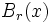# Injectivity radius

(diff) ← Older revision | Latest revision (diff) | Newer revision → (diff)
Jump to: navigation, search

This article defines a scalar function on a manifold, viz a function from the manifold to real numbers. The scalar function may be intrinsic or defined in terms of some other structure/functionsTemplate:Radius notion

The term injectivity radius is also used for injectivity radius of a manifold which is the infimum over the manifold of the injectivity radii at all points

## Definition

The injectivity radius is a scalar function on a Riemannian manifold$M$ is defined as follows: the injectivity radius at$x \in M$ is the supremum of all values$r$ such that the exponential map from the unit ball$B_r(x)$ in$T_xM$, to the manifold$M$, is injective.

The fact that the injectivity radius at each point is strictly positive is one of the starting points of Riemannian geometry.